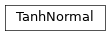# garage.torch.distributions¶

PyTorch Custom Distributions.

class TanhNormal(loc, scale)

Bases: torch.distributions.DistributionA distribution induced by applying a tanh transformation to a Gaussian random variable.

Algorithms like SAC and Pearl use this transformed distribution. It can be thought of as a distribution of X where

$$Y ~ \mathcal{N}(\mu, \sigma)$$ $$X = tanh(Y)$$

Parameters
• loc (torch.Tensor) – The mean of this distribution.

• scale (torch.Tensor) – The stdev of this distribution.

log_prob(self, value, pre_tanh_value=None, epsilon=1e-06)

The log likelihood of a sample on the this Tanh Distribution.

Parameters
• value (torch.Tensor) – The sample whose loglikelihood is being computed.

• pre_tanh_value (torch.Tensor) – The value prior to having the tanh function applied to it but after it has been sampled from the normal distribution.

• epsilon (float) – Regularization constant. Making this value larger makes the computation more stable but less precise.

Note

when pre_tanh_value is None, an estimate is made of what the value is. This leads to a worse estimation of the log_prob. If the value being used is collected from functions like sample and rsample, one can instead use functions like sample_return_pre_tanh_value or rsample_return_pre_tanh_value

Returns

The log likelihood of value on the distribution.

Return type

torch.Tensor

sample(self, sample_shape=torch.Size())

Return a sample, sampled from this TanhNormal Distribution.

Parameters

sample_shape (list) – Shape of the returned value.

Note

Gradients do not pass through this operation.

Returns

Sample from this TanhNormal distribution.

Return type

torch.Tensor

rsample(self, sample_shape=torch.Size())

Return a sample, sampled from this TanhNormal Distribution.

Parameters

sample_shape (list) – Shape of the returned value.

Note

Returns

Sample from this TanhNormal distribution.

Return type

torch.Tensor

rsample_with_pre_tanh_value(self, sample_shape=torch.Size())

Return a sample, sampled from this TanhNormal distribution.

Returns the sampled value before the tanh transform is applied and the sampled value with the tanh transform applied to it.

Parameters

sample_shape (list) – shape of the return.

Note

Returns

Samples from this distribution. torch.Tensor: Samples from the underlying

torch.distributions.Normal distribution, prior to being transformed with tanh.

Return type

torch.Tensor

cdf(self, value)

Returns the CDF at the value.

Returns the cumulative density/mass function evaluated at value on the underlying normal distribution.

Parameters

value (torch.Tensor) – The element where the cdf is being evaluated at.

Returns

the result of the cdf being computed.

Return type

torch.Tensor

icdf(self, value)

Returns the icdf function evaluated at value.

Returns the icdf function evaluated at value on the underlying normal distribution.

Parameters

value (torch.Tensor) – The element where the cdf is being evaluated at.

Returns

the result of the cdf being computed.

Return type

torch.Tensor

expand(self, batch_shape, _instance=None)

Returns a new TanhNormal distribution.

(or populates an existing instance provided by a derived class) with batch dimensions expanded to batch_shape. This method calls expand on the distribution’s parameters. As such, this does not allocate new memory for the expanded distribution instance. Additionally, this does not repeat any args checking or parameter broadcasting in __init__.py, when an instance is first created.

Parameters
• batch_shape (torch.Size) – the desired expanded size.

• _instance (instance) – new instance provided by subclasses that need to override .expand.

Returns

New distribution instance with batch dimensions expanded to batch_size.

Return type

Instance

enumerate_support(self, expand=True)

Returns tensor containing all values supported by a discrete dist.

The result will enumerate over dimension 0, so the shape of the result will be (cardinality,) + batch_shape + event_shape (where event_shape = () for univariate distributions).

Note that this enumerates over all batched tensors in lock-step [[0, 0], [1, 1], …]. With expand=False, enumeration happens along dim 0, but with the remaining batch dimensions being singleton dimensions, [, , ...

To iterate over the full Cartesian product use itertools.product(m.enumerate_support()).

Parameters

expand (bool) – whether to expand the support over the batch dims to match the distribution’s batch_shape.

Note

Calls the enumerate_support function of the underlying normal distribution.

Returns

Tensor iterating over dimension 0.

Return type

torch.Tensor

property mean(self)

torch.Tensor: mean of the distribution.

property variance(self)

torch.Tensor: variance of the underlying normal distribution.

entropy(self)

Returns entropy of the underlying normal distribution.

Returns

entropy of the underlying normal distribution.

Return type

torch.Tensor